# GSEB Solutions Class 10 Maths Chapter 6 Triangle Ex 6.4

Gujarat Board GSEB Solutions Class 10 Maths Chapter 6 Triangle Ex 6.4 Textbook Questions and Answers.

## Gujarat Board Textbook Solutions Class 10 Maths Chapter 6 Triangle Ex 6.4

Question 1.
Let ∆ABC ~ ∆DEF and their areas be, respectively 64 cm2 and 121 cm2. If EF = 15.4 cm, find BC.
Solution:
We have
∆ABC ~ ∆DEF
ar ∆ABC = 64 cm2
ar ∆DEF = 14cm2
and EF = 15.4 cm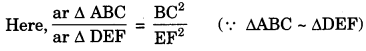⇒ $$\frac {64}{121}$$ = $$\frac{\mathrm{BC}^{2}}{(15.4)^{2}}$$
⇒ $$\left(\frac{8}{11}\right)^{2}$$ = $$\left(\frac{\mathrm{BC}}{15.4}\right)^{2}$$
⇒ $$\frac {8}{11}$$ = ⇒ $$\frac {BC}{15.4}$$
(Taking square root on both sides)
⇒ BC = $$\frac {8}{11}$$ x 15.4
BC = 11.2cmQuestion 2.
Diagonals of a trapezium ABCD with AB DC intersect each other at the point O. If AB = 2 CD, find the ratio of the areas of triangles AOB and COD. (CBSE 2012)
Solution:
Trapezium ABCD, in which AB DC and diagonal AC and BD intersect at O.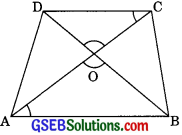In ∆AOB and ∆COD
∠AOB = ∠COD (Vertically opposite angle)
∠OAB = ∠OCD (Alternate Interior angles)
∴ ∆AOB ~ ∆COD (by AA similarity)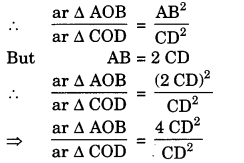∴ar ∆AOB: ar ∆COD = 4: 1

Question 3.
In Figure, ABC and DBC are two triangles on the same base BC. If AD intersects BC at O, arAABC AO
show that =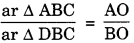(CBSE 2005, 2012)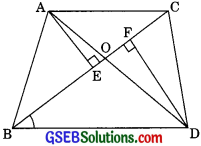Solution:
∆ABC and IDBC are two triangles on the same base BC and diagonals AD and BC Intersect each other at O.
Let us draw AE ⊥ BC and DF ⊥ BC
In ∆AOE and ∆DOF
∠AOE = ∠DOF (Vertically opposite angles)
∠AEO = ∠DFO (each 900)
∆AOE ~ ∆DOF (by AA similarity)
$$\frac {AE}{DF}$$ = $$\frac {AO}{DO}$$ ………(1)
(Corresponding sides of two similar triangles are proportional)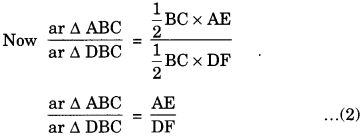From equations (1) and (2)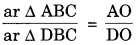Question 4.
If the area of two similar triangles are equal, prove that they are congruent. (CBSE 2012)
Solution:
Given: ∆ABC and ∆DEF are two similar triangles such that, ar ∆ABC = ar ∆DEF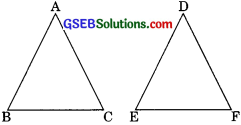To prove: ∆ABC ≅ ∆DEF
Proof: ∆ABC ~ ∆DEF (given)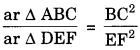(The ratio of the areas of two similar triangles is equal to the square of the ratio of their corresponding sides)
1 = $$\frac{\mathrm{BC}^{2}}{\mathrm{EF}^{2}}$$ (ar ∆ABC = ar ∆DEF)
EF2 = BC2
BC = EF
Also ∠ABC = ∠DEF
and ∠ACB = ∠DFE
∴∆ABC ≅ ∆DEF (by ASA congruency criterion)Question 5.
D, E and F are respectively the midpoints of sides AB, BC and CA of ∆ABC. Find the ratio of the area of ∆DEF and ∆ABC.
Solution:
D, E and F are respectively the midpoints of the sides of ∆ABC. Jour the points D, E and F to form ∆DEF.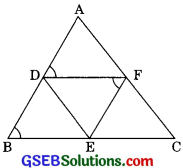We have = $$\frac{AD}{AB}$$ = $$\frac{1}{2}$$ ……..(1)
(D is the mid point of AB)
and $$\frac{AF}{AC}$$ = $$\frac{1}{2}$$ ………(2)
(F is the mid point of AC)
From eqn (1) and (2)
We have $$\frac{AD}{AB}$$ = $$\frac{AF}{AC}$$
∴ DF || BC (by converse of BPT)
and ∆AFD = ∆ACE ……….(4)
(corresponding angles)
∴ ∆DEF ~ ∆ABC (by AA similarity)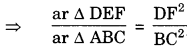(The ratio of the areas of two similar triangles is equal to the square of the ratio of their corresponding sides)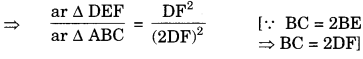Line drawn from mid points of two sides of a triangle is parallel to third and half of the third.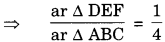ar ∆DEF : ar ∆ABC = 1 : 4Question 6.
Prove that the ratio of the areas of two similar triangles is equal to the square of the ratio of their corresponding medians.
Solution:
Given: ∆ABC and ∆DEF such that
∆ABC ~ ∆DEF
AM and DN are their corresponding medians.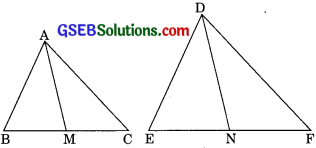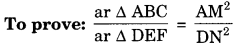Proof: ∆ABC ~ ∆DEF (given)
∴ $$\frac{AB}{DE}$$ = $$\frac{BC}{EF}$$ = $$\frac{AC}{DF}$$ ……..(1)
(corresponding sides of two similar triangles are proportional)
or $$\frac{AB}{DE}$$ = $$\frac{2BC}{2EN}$$ (AM and DN are medians)
$$\frac{AB}{DE}$$ = $$\frac{BM}{EN}$$ ………(2)
and ∠ABM = ∠DEN
(corresponding angles of similar triangles)
∴ ∠ABM – ∠ADEN (by SAS similarity)
$$\frac{AB}{DE}$$ = $$\frac{BM}{EN}$$ = $$\frac{AM}{DN}$$ ………(3)
(corresponding sides of the similar triangles are proportional)
From eqn (2) and (3) we get
$$\frac{AB}{DE}$$ = $$\frac{AM}{DN}$$ ………(4)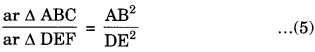(Area of two similar triangles is equal to square of the ratio of their corresponding sides)
From eqn (4) and (5)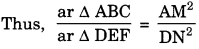Question 7.
Prove that the area of an equilateral triangle described on one side of a square is equal to half the area of the equilateral triangle described on one of its diagonals. (CBSE 2012)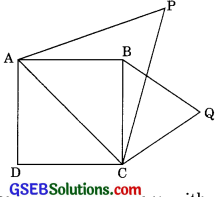Solution:
Given: ABCD is a square with diagonal AC and equilateral ZBQC is described on the side BC and another equilateral AAPC is described on the diagonal AC.
To prove: ar ∆BQC = $$\frac{1}{2}$$ ar ∆APC
Proof: ∆APC ~ ∆BQC (by AAA similarity)
(Both triangles are equilateral)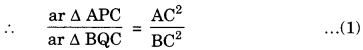(The ratio of areas of two similar triangles is equal to the square of their corresponding sides)
AC = $$\sqrt {2}$$BC ………(2)
From eqn (1) and (2)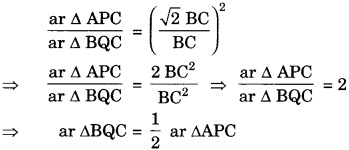Question 8.
ABC and BDE are two equilateral triangles such that D is the midpoint of BC. The ratio of the areas of triangles ABC and BDE
(a) 2 : 1
(b) 1 : 2
(c) 4 : 1
(d) 1 : 4
Solution:
∆ABC and ∆BDE are both equilateral triangles
∴ ∆ABC ~ ∆BDE (by AAA similarity)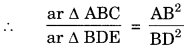(Ratio of areas of two similar triangles is equal to the square of the ratio of their corresponding sides)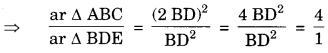(D is the mid point of BC and AB = BC = CA)
∴ar ∆ABC : ar ∆BDE = 4 : 1
Thus correct option is (c).Question 9.
Sides of two similar triangles are in the ratio 4: 9. The area of these triangles are in the ratio.
(a) 2 : 3
(b) 4 : 9
(c) 81 : 16
(d) 16 : 81
Solution:
Let ∆ABC and ∆DEF are similar then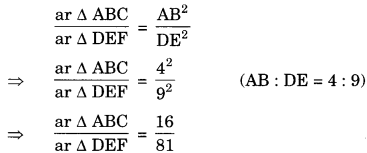ar ∆ABC : ar ∆DEF = 16 : 81
Thus (d) is the correct answer.